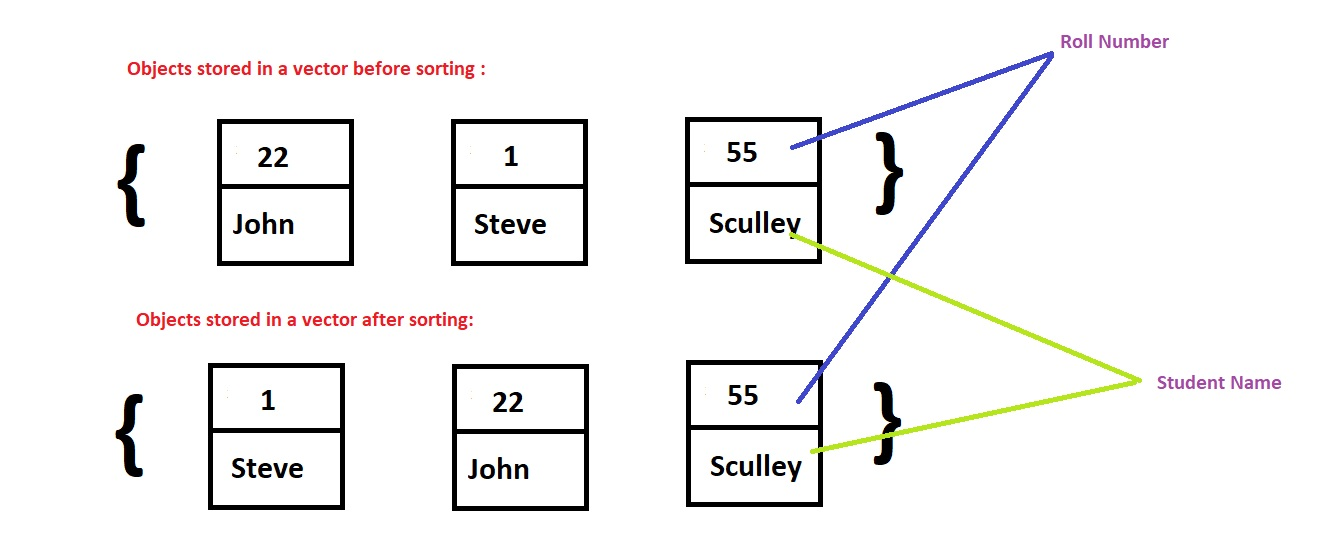×

Search anything:

# Sort Vector of Custom Object in C++ STL

#### C++Get this book -> Problems on Array: For Interviews and Competitive Programming

In this article, we will sort a vector having custom object as elements of vector in C++ using comparator.

1. Explanation
2. Create a Class
3. Sort function
4. Comparator function
5. Code
6. Input/Output
7. Time & Space Complexity

## Explanation

Suppose we are given an object named Student. It stores the name of Student and the Roll number. All the object is stored in a vector randomly. We are required to sort the vector in terms of increasing Roll number.## Create a Object

We create a custom Object with the name "Student". It has two data members : Roll Number and Student Name, which store the name of each student and his/her roll number.

``````struct Student {
int rollNumber;
string stu_Name;
Student(int rollNumber, string stu_Name) {
this->rollNumber = rollNumber;
this->stu_Name   = stu_Name;
}
};
``````

### Sort function

In this program we will use the sort function having three parameter.

sort(first-iterator, second-iterator, comparator-function)

• first-iterator : This iterator points to the starting address of a cointainer.
• second-iterator : This iterator points to the next to last address of a container.
• comparator-function : This function decide in which order we want to sort our container.It returns boolean result i.e true or false.

### Comparator Function

The idea of comparator function is as follows:

``````comparator(obj1, obj2)
return X
``````
• If X < 0, then obj1 is placed before obj2
• If X = 0, then obj1 and obj2 have equal preference
• If X > 0, then obj1 is placed after obj2.

So, accordingly the comparator can be set.

This function return boolean result i.e true or false.

• Increasing Order: If we want to arrange elements in Increasing order than we write the code as-
``````bool comparator(const Student& s1, const Student& s2) {
return s1.rollNumber - s2.rollNumber;
}
``````

If roll number of student at OpenGenus s1 is < s2, the function will return a negative value so s1 will be placed before s2.

• Decreasing Order: If we want to arrange elements in Decreasing order than we write the code as-
``````bool comparator(const Student& s1, const Student& s2) {
return s2.rollNumber - s1.rollNumber;
}
``````

If roll number of student s1 is < s2, the function will return a positive value so s1 will be placed after s2.

Note: Here we have arranged in terms of Roll Number. But if you want to arrange in terms of other data member than you can do that by replacing rollNumber with any other type of data member that you have initialised in the object.
Example: You have a data member "percentage" in your Object Student and you want to arrange in Decreasing order then just replace s1.rollNumber with s1.percentage and s2.rollNumber with s2.percentage.

If there is a tie in percentage, one can check rollNumber as second sorting parameter as follows:

``````bool comparator(const Student& s1, const Student& s2) {
double return_val = s2.percentage - s1.percentage;
if (return_val == 0)
return s1.rollNumber - s2.rollNumber
}
``````

This is sorting in:

• Descending order of percentage
• If percentage is same, then ascending order of roll number

### Code

Following is the complete C++ implementation using the concept:

``````#include<bits/stdc++.h>

using namespace std;

// Custom Object
struct Student {
int rollNumber;
string stu_Name;
Student(int rollNumber, string stu_Name) {
this->rollNumber = rollNumber;
this->stu_Name   = stu_Name;
}
};

//Comparator function
bool comparator(const Student& s1, const Student& s2) {
return s1.rollNumber < s2.rollNumber;
}

int main() {

vector<Student> v;

int n;
cout<<"Enter number of students data you want to enter : "<<endl;
cin>>n;

for(int i=0;i<n;i++) {
int rollNumber;
string stu_Name;
cout<<"Enter Roll Number:"<<endl;
cin>>rollNumber;
cout<<"Enter Student Name: "<<endl;
cin>>stu_Name;
cout<<endl;
v.push_back(Student(rollNumber, stu_Name));
}

// Sorting the vector
sort(v.begin(), v.end(), &comparator);

for(auto i:v) {
cout<<i.rollNumber<<' '<<i.stu_Name<<endl;
}

return 0;
}
``````

### Input/Output

INPUT :

``````Enter number of students data you want to enter :
3
Enter Roll Number:
22
Enter Student Name:
John

Enter Roll Number:
1
Enter Student Name:
Steve

Enter Roll Number:
55
Enter Student Name:
Sculley

``````

OUTPUT :

``````1 Steve
22 John
55 Sculley
``````

### Time & Space Complexity

TIME COMPLEXITY : O(nlogn)
SPACE COMPLEXITY : O(1)